Distance Speed Time Formula - www.studyandupdates.com

## Distance Speed Time Formula

Speed is a measure of how quickly an object moves from one place to another. It is equal to the distance traveled divided by the time. It is possible to find any of these three values using the other two. This picture is helpful:The positions of the words in the triangle show where they need to go in the equations. To find the speed, distance is over time in the triangle, so speed is distance divided by time. To find distance, speed is beside time, so distance is speed multiplied by time.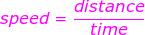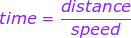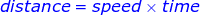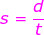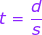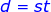s = speed (meters/second)
d = distance traveled (meters)
t = time (seconds)